# MROUND In ExcelArticle byExcelMojo Team## What Is MROUND Function In Excel?

The MROUND function in Excel is an inbuilt Math & Trig function that returns a number rounded to a multiple specified by the user. Users can use the function MROUND in Excel to remove the least significant digits in a number. Thus, it helps make the number appear more presentable while remaining close to the value in the source data.

For example, the following table contains a list of numbers and the multiples to which we require to round them.

And suppose we need to display the results in column C cells, C2:C7. Then we can achieve the required outcome using MROUND in Excel.

The above MROUND in Excel example shows each number gets rounded up or down, depending on the nearest multiple. And the resulting rounded numbers appear more readable than column A numbers. Thus, MROUND in Excel indeed makes the numbers easier to read.

###### Key Takeaways
• The function MROUND in Excel returns a numeric value rounded to a specified multiple. Thus, users can use it to remove the least significant digits from numbers, such as currencies, making them easier to read while being close to the source data.
• The MROUND() accepts two mandatory arguments, number and multiple, as input.
• The function MROUND() rounds up the supplied number if the remainder of dividing it by the given multiple is equal to or more than half the multiple. Otherwise, it rounds down the given numeric value.

### MROUND() Excel Formula

The syntax for MROUND formula in Excel syntax is:

where,

• number: The value we require to round.
• multiple: The multiple to which we require to round the specified number.

The above two arguments in the MROUND formula in Excel are mandatory. While we can directly enter the two arguments as numbers, we can supply them as Excel cell references to the specific numbers, as depicted in the previous section.

Please Note: If we require to round a time value to the nearest time multiple, say minutes, provide the argument multiple to the MROUND() as a time value in double quotations.

Below are a few critical aspects of MROUND in Excel we must consider to avoid potential errors.

• Both the arguments, number and multiple, should be numeric values. Otherwise, the MROUND() returns the #NAME? error.
• The two arguments, number and multiple should be of the same sign. Otherwise, the MROUND in Excel return value will be the #NUM! error.
• Suppose the remainder we get from dividing the given number by the specified multiple is equal to or more than half the multiple. Then the function MROUND in Excel rounds up the given number to the nearest multiple. Otherwise, the number gets rounded down to the nearest multiple.

### How to Use MROUND Excel Function?

The steps to use MROUND() are:

1. First, ensure the source data contains numeric values.
2. Then select the target cell and enter the function MROUND in Excel. Ensure the arguments we supply are of the same sign.
3. Finally, press Enter to view the required outcome.

Below is an MROUND example to explain the above steps.

Suppose the table below shows ten consecutive numbers, and we must round them to the nearest multiple of 5.

Then, using MROUND in Excel, we can populate column C with the desired rounded numbers.

1. Select the target cell C2, enter the following MROUND(), and press Enter.

=MROUND(A2,5)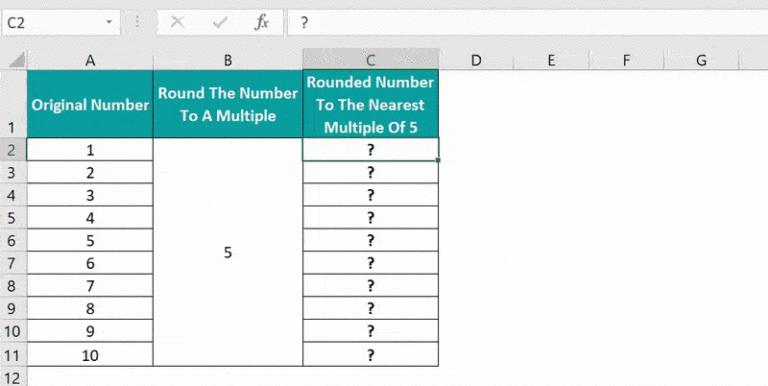Alternatively, we can select the target cell C2 and click FormulasMath & TrigMROUND to open the Function Arguments window.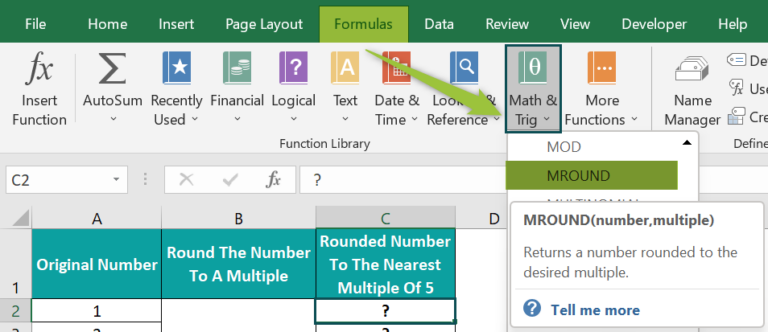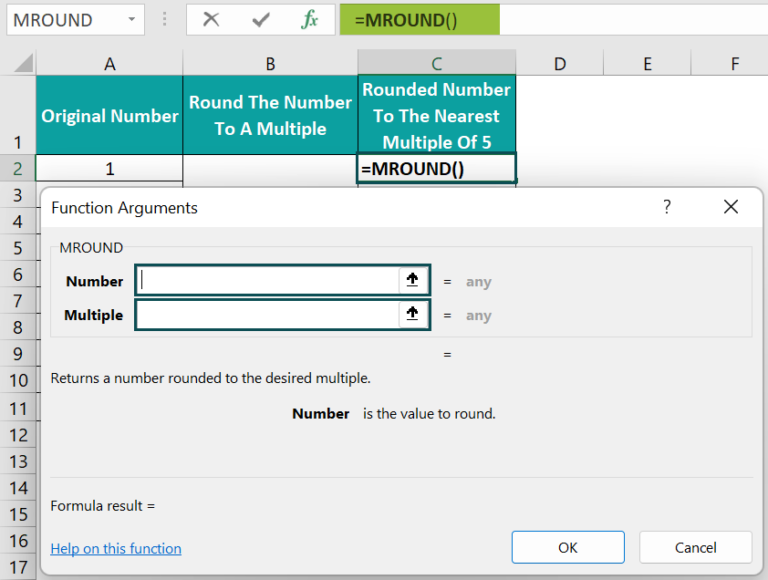Enter the two argument values in the Function Arguments window.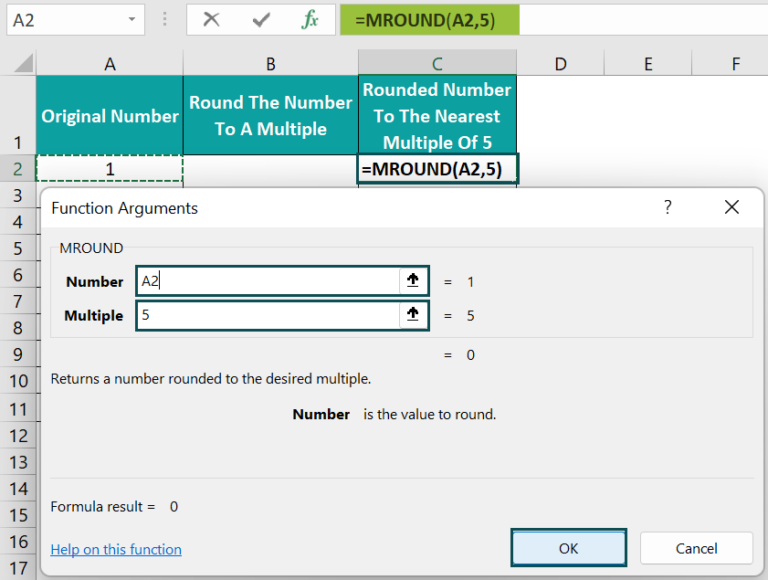And once we click OK, we can see the result 0 in the target cell C2.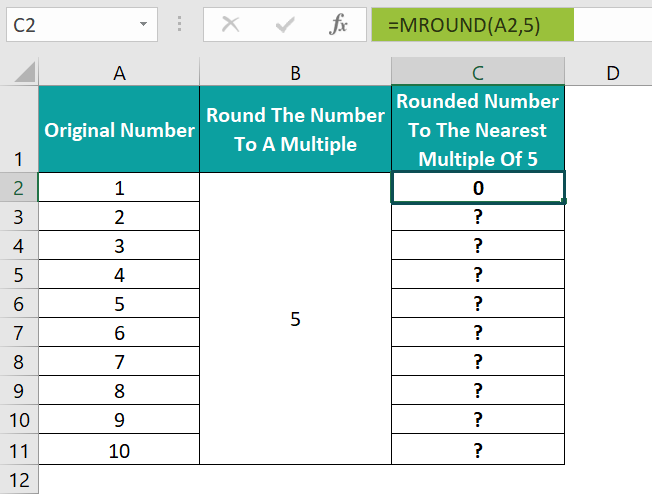2. Drag the fill handle downwards to copy the formula in cell range C3:C11.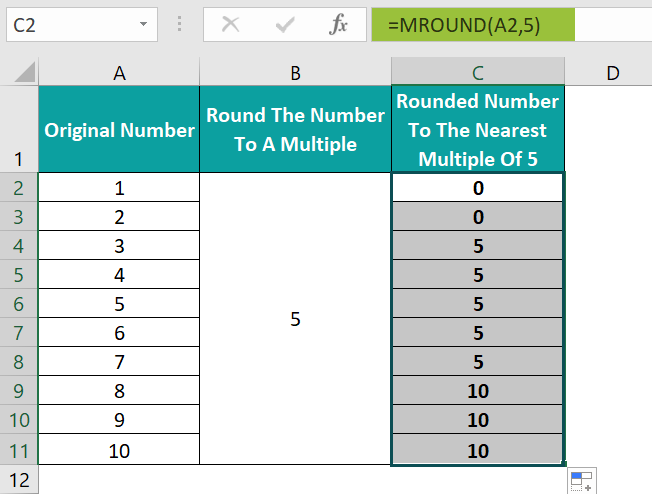In this example, the first argument in the MROUND(), number, is a cell reference to the original number. On the other hand, we provide the second argument directly as a numeric value to the MROUND().

We can also enter the arguments as depicted below. Let us consider the formula in the target cell C11.
=MROUND(10,5) or =MROUND(A11,B2)

The MROUND in Excel return value will be the same in each case, 10.

### Examples

Below are a few more examples to effectively understand the function MROUND in Excel.

#### Example #1

Assume we have a list of fruits and their required quantities.

Also, the table includes the data regarding the available stock per unit.

Suppose we require to round the quantities to the multiples of the stock per unit values so that placing their orders will be more straightforward. Then, we can apply the function MROUND in the target cells in column D to get the required rounded quantities.

• Step 1: Select the target cell D2, enter the MROUND() provided in the Formula Bar in the below image, and press Enter.
• Step 2: Drag the fill handle downwards to copy the formula for MROUND in Excel in cells D3:D6.

While the MROUND() rounded up the quantity values for the fruits in cells A2:A4, it rounded down the quantity values for Pear and Mango.

The reason is that, for instance, the nearest multiples of 20 to 148 are 140 and 160 in the case of Mango. And between the two, 140 is closest to 148. Also, the remainder of dividing 148 by 20 is 1, which is lower than half of 20. Thus, it gets rounded down.

So, now we can place the order for each fruit as an integer or multiple of the unit, making the process less complicated.

#### Example #2

Let us see how the function MROUND in Excel behaves when the supplied arguments are decimal values.

Consider the below table. It contains a list of office stationery items and their prices.

Suppose we need to round the price values to the specified multiples and display the output in the target cell range D2:D6. Then we can apply the function MROUND to the target cells in the following way.

• Step 1: Select the target cell D2, enter the MROUND() provided in the Formula Bar in the below image, and press Enter.
• Step 2: Drag the fill handle downwards to copy the formula in cell range D3:D6.

Thus, now the rounded price value of each item is more presentable.

#### Example #3

This example shows how the function MROUND works while rounding time values.

Assume the following table shows a list of time values, and we require to round them to 15 minutes and 1 hour.

Please Note: The cell range A2:C6 have the data format set as Custom, h:mm:ss AM/PM in the Home tab. We can click Number Format More Number Formats in the Home tab to open the Format Cells window.

Then, in the Format Cells window, go to the Number tab, click Custom and pick the required time format.

We can use the function MROUND in Excel to populate the required data in the target columns B and C.

• Step 1: Select the target cell B2, enter the following MROUND(), and press Enter.

=MROUND(A2,”0:15″)

• Step 2: Drag the fill handle downwards to copy the formula in the range B3:B6.
• Step 3: Select the target cell C2, enter the following MROUND(), and press Enter.

=MROUND(A2,”1:00″)

• Step 4: Drag the fill handle downwards to copy the formula for MROUND in Excel in cells C3:C6.

Thus, the MROUND() rounds the time values to the nearest multiples of 15 minutes and 1 hour, applying the same logic as explained in the previous examples.

### Important Things to Note

• Ensure we supply numeric values or cell references to numeric values as arguments to the MROUND(). Otherwise, the function output will be the #NAME? error.
• Ensure the two arguments have the same sign to avoid the function MROUND returning the  #NUM! error.
• While rounding a time value using the MROUND() to the nearest time multiple, such as minutes, provide the argument multiple as a time value in double quotations.
• The MROUND() can round up or down positive and negative integers, decimal values, and time values.

1. Where is the MROUND function in Excel?

The MROUND function in Excel is in the Formulas tab. Click FormulasMath & Trig MROUND to access it.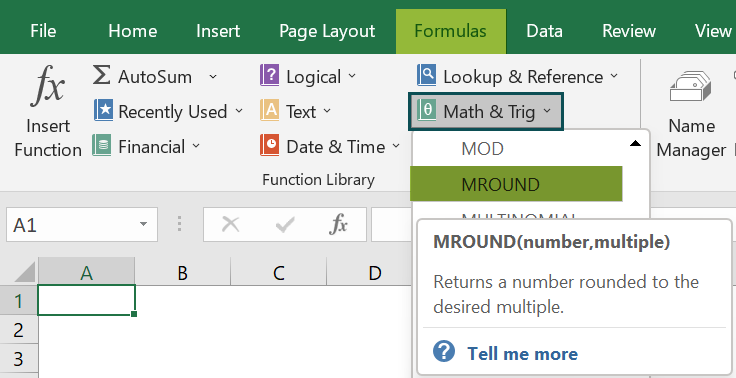2. Does MROUND in Excel round up or down?

The MROUND in Excel rounds up or down the given number depending on the nearest multiple.
If the remainder of dividing the given number by the specified multiple is equal to or more than half of the multiple, the function rounds up the number away from 0. Otherwise, it rounds down the specified number.

3. When to use MROUND in Excel?

We can use MROUND in Excel when removing the least significant digits from numeric values, such as currencies and time values, to make them more presentable.
And we can use the function when we require a number rounded to a specific multiple.

4. How do you do MROUND in Excel with negative numbers?

We can do MROUND in Excel with negative numbers in the following way. Let us see the steps with an example.
Suppose the table below contains negative temperature values in column A and we have to round them up to the negative multiples specified in column B.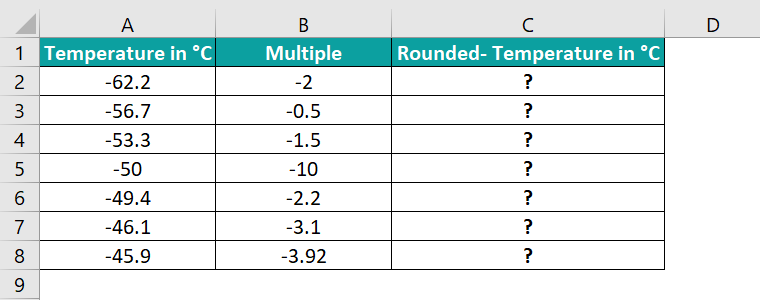We can apply the MROUND() in the target cell range C2:C8 to achieve the required rounded negative temperature values. And the steps are:

• Step 1: Select the target cell C2, enter the MROUND() provided in the Formula Bar in the below image, and press Enter.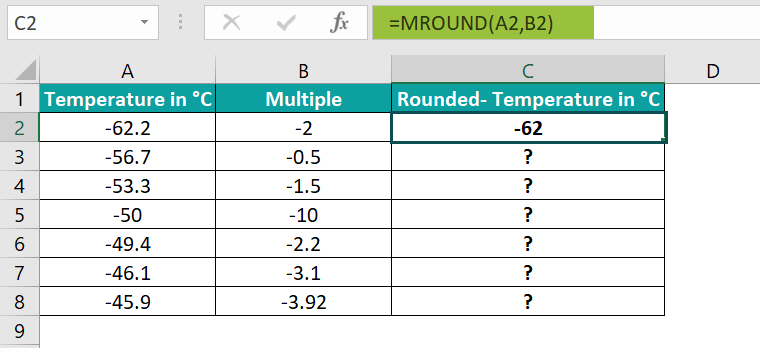• Step 2: Drag the fill handle downwards to copy the formula in cell range C3:C8.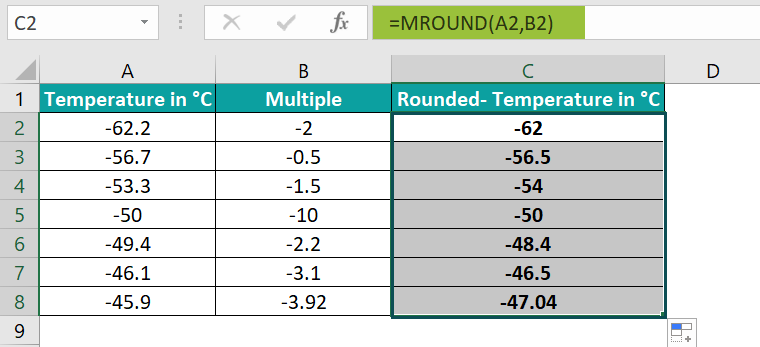Thus, we can use the MROUND() with negative numbers. The only condition is that both the arguments we provide to the function should be negative.